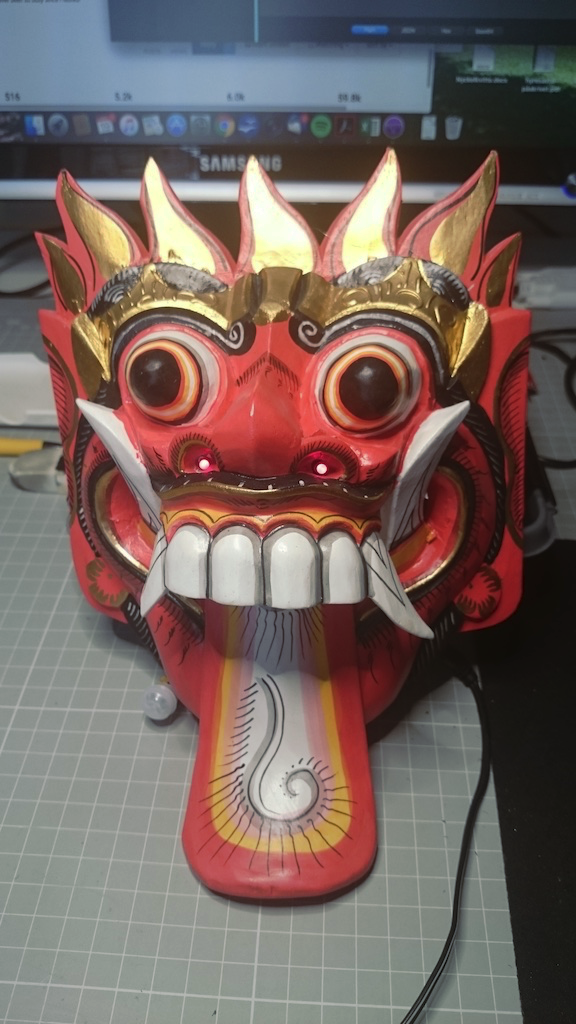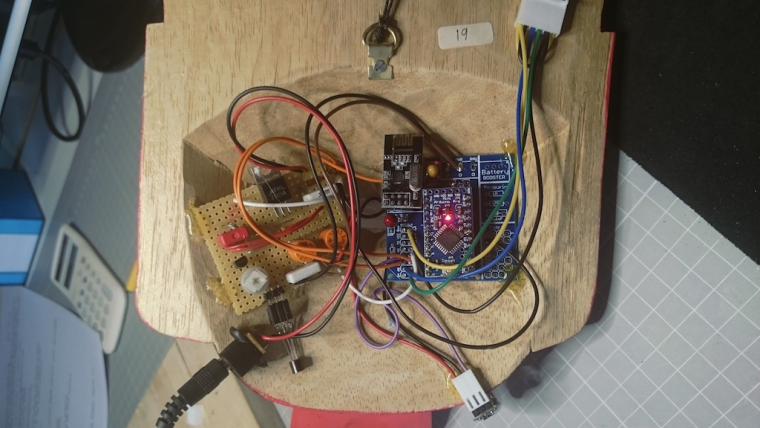# Motion detector with temperature and barometer function

• I have built an intrusion detector that also measures barometer pressure and indoor and outdoor temperatures.

The pressure and indoor temperature is measured by a BMP280 and a number of DS18B20 sensors can be connected to measure the temperature in remote locations.

When movement is detected two red LEDs flash during 20 sec.

Everything is built into a frightening wooden mask, bought in Indonesia. The LEDs poke out through the nostrils.

Here is the sketch:

``````/**
* File name: MotionSensor_LED_2_temp_baro_280
* The MySensors Arduino library handles the wireless radio link and protocol
* between your home built sensors/actuators and HA controller of choice.
* The sensors forms a self healing radio network with optional repeaters. Each
* repeater and gateway builds a routing tables in EEPROM which keeps track of the
* network topology allowing messages to be routed to nodes.
*
* Copyright (C) 2013-2015 Sensnology AB
* Full contributor list: https://github.com/mysensors/Arduino/graphs/contributors
*
* Documentation: http://www.mysensors.org
* Support Forum: http://forum.mysensors.org
*
* This program is free software; you can redistribute it and/or
* modify it under the terms of the GNU General Public License
*
*******************************
*
* REVISION HISTORY
* Version 1.0 - Henrik Ekblad
* Version 2.1 - Gunnar Blockmar includes PMB280 temp/baro sensor and DS18B20 temp sensor
*
* DESCRIPTION
* Motion Sensor example using HC-SR501
* http://www.mysensors.org/build/motion
*
* Now with 1 fading LEDs when sensor is triggered
* Measuring temp and pressure with BMP 280. Local pressure
* Measuring remote temp via DS18B20
*/

#include <OneWire.h>
#include <DallasTemperature.h>
#include <SPI.h>

unsigned long SLEEP_TIME = 60000; // Sleep time between reports (in milliseconds)

#define MY_DEBUG
#define DIGITAL_INPUT_SENSOR 3   // The digital input you attached your motion sensor.  (Only 2 and 3 generates interrupt!)
#define INTERRUPT DIGITAL_INPUT_SENSOR-2 // Usually the interrupt = pin -2 (on uno/nano anyway)
#define CHILD_ID_MOT 0   // Id of the motion sensor child
#define CHILD_ID_ITEMP 1  // Id of indoor temperature sensor
#define CHILD_ID_BARO 2  // Id of pressure sensor
#define CHILD_ID_OTEMP 3  // Id of outdoor temperature sensor
#define RED_LED 5    // Pin for LED
#define RED_LED2 6  // Pin for LED2
#define DELAY 40     // Wait for brightness change
int fadeAmount = 5;   // brightness change per step
#define ONE_WIRE_BUS 2 // Pin where dallas sensor is connected
#define MAX_ATTACHED_DS18B20 16
OneWire oneWire(ONE_WIRE_BUS); // Setup a oneWire instance to communicate with any OneWire devices (not just Maxim/Dallas temperature ICs)
DallasTemperature sensors(&oneWire); // Pass the oneWire reference to Dallas Temperature.
int numSensors=0;

const char *weather[] = { "Stabilt", "Bättre", "Sämre", "Ostadigt", "Åska!", "Okänt" };
enum FORECAST
{
STABLE = 0,     // "Stable Weather Pattern"
SUNNY = 1,      // "Slowly rising Good Weather", "Clear/Sunny "
CLOUDY = 2,     // "Slowly falling L-Pressure ", "Cloudy/Rain "
UNSTABLE = 3,   // "Quickly rising H-Press",     "Not Stable"
THUNDERSTORM = 4, // "Quickly falling L-Press",    "Thunderstorm"
UNKNOWN = 5     // "Unknown (More Time needed)
};

Adafruit_BMP280 bmp;      // Digital Pressure Sensor

float lastPressure = -1;
float lastTemp = -1;
int lastForecast = -1;

const int LAST_SAMPLES_COUNT = 5;
float lastPressureSamples[LAST_SAMPLES_COUNT];

// this CONVERSION_FACTOR is used to convert from Pa to kPa in forecast algorithm
// get kPa/h by dividing hPa by 10
#define CONVERSION_FACTOR (1.0/10.0)

int minuteCount = 0;
bool firstRound = true;
// average value is used in forecast algorithm.
float pressureAvg;
// average after 2 hours is used as reference value for the next iteration.
float pressureAvg2;
float dP_dt;

// Enable debug prints to serial monitor
// #define MY_DEBUG

// Enables and select radio type (if attached)

#define MY_NODE_ID 41

#include <MySensors.h>

// Initialize motion message
MyMessage msgm(CHILD_ID_MOT, V_TRIPPED);
// Initialize temp baro and forecast messages
MyMessage msgit(CHILD_ID_ITEMP, V_TEMP);
MyMessage msgp(CHILD_ID_BARO, V_PRESSURE);
MyMessage forecastMsg(CHILD_ID_BARO, V_FORECAST);
MyMessage msgot(0, V_TEMP);

bool metric = true;
void before()
{
// Startup up the OneWire library
sensors.begin();
}

void setup()
{
pinMode(DIGITAL_INPUT_SENSOR, INPUT);      // sets the motion sensor digital pin as input
pinMode(RED_LED, OUTPUT);   // sets the pin for the LEDs as output
pinMode(RED_LED2, OUTPUT);
analogWrite(RED_LED, 0);    // make sure LEDs are OFF
analogWrite(RED_LED2, 0);
bmp.begin();
if (!bmp.begin())
{
Serial.println("Could not find a valid BMP280 sensor, check wiring!");
while (1) {}
}
// requestTemperatures() will not block current thread
sensors.setWaitForConversion(false);
}

void presentation()
{
// Send the sketch version information to the gateway and Controller
sendSketchInfo("M. Sens_LED_2t/b/f 280", "2.2");

// Fetch the number of attached temperature sensors
numSensors = sensors.getDeviceCount();

// Register all sensors to gw (they will be created as child devices)
present(CHILD_ID_MOT, S_MOTION);
present(CHILD_ID_ITEMP, S_TEMP);
present(CHILD_ID_BARO, S_BARO);
for (int i=0; i<numSensors && i<MAX_ATTACHED_DS18B20; i++) {
present(i+CHILD_ID_OTEMP, S_TEMP);
}
}

void loop()
{

boolean tripped = digitalRead(DIGITAL_INPUT_SENSOR) == HIGH;

Serial.println(tripped);
send(msgm.set(tripped?"1":"0"));  // Send tripped value to gw

if (tripped) {
int brightness = 0;   // brightness of LED
int count = 0;  // number of half cycles to fade LED
while (count < 12) {    // run 6 full fade cycles
analogWrite(RED_LED, brightness);
analogWrite(RED_LED2, brightness);
if (brightness == 0 || brightness == 255) {
count ++;
}
wait (DELAY);  //wait to see brightness change
}
analogWrite(RED_LED, 0);  // turn off LEDs
analogWrite(RED_LED2, 0);
}

int long pressure = bmp.readPressure() / 100;
int forecast = sample(pressure);

send(msgit.set(temperature, 1));
send(msgp.set(pressure, 0));
send(forecastMsg.set(weather[forecast]));

// Fetch temperatures from Dallas sensors
sensors.requestTemperatures();

sleep (750);

// Read temperatures and send them to controller
for (int i=0; i<numSensors && i<MAX_ATTACHED_DS18B20; i++) {
// Fetch and round temperature to one decimal
float otemperature = static_cast<float>(static_cast<int>((sensors.getTempCByIndex(i)) * 10.)) / 10.;

// Only send data if no error

if (otemperature != -127.00 && otemperature != 85.00) {
// Send in the new temperature
send(msgot.setSensor(i+CHILD_ID_OTEMP).set(otemperature,1));
}
}

// Sleep until interrupt comes in on motion sensor but send update every minute.
tripped = false;  //reset tripped
sleep(INTERRUPT,CHANGE, SLEEP_TIME);
}

float getLastPressureSamplesAverage()
{
float lastPressureSamplesAverage = 0;
for (int i = 0; i < LAST_SAMPLES_COUNT; i++)
{
lastPressureSamplesAverage += lastPressureSamples[i];
}
lastPressureSamplesAverage /= LAST_SAMPLES_COUNT;

return lastPressureSamplesAverage;
}

// Algorithm found here
// http://www.freescale.com/files/sensors/doc/app_note/AN3914.pdf
// Pressure in hPa -->  forecast done by calculating kPa/h
int sample(float pressure)
{
// Calculate the average of the last n minutes.
int index = minuteCount % LAST_SAMPLES_COUNT;
lastPressureSamples[index] = pressure;

minuteCount++;
if (minuteCount > 185)
{
minuteCount = 6;
}

if (minuteCount == 5)
{
pressureAvg = getLastPressureSamplesAverage();
}
else if (minuteCount == 35)
{
float lastPressureAvg = getLastPressureSamplesAverage();
float change = (lastPressureAvg - pressureAvg) * CONVERSION_FACTOR;
if (firstRound) // first time initial 3 hour
{
dP_dt = change * 2; // note this is for t = 0.5hour
}
else
{
dP_dt = change / 1.5; // divide by 1.5 as this is the difference in time from 0 value.
}
}
else if (minuteCount == 65)
{
float lastPressureAvg = getLastPressureSamplesAverage();
float change = (lastPressureAvg - pressureAvg) * CONVERSION_FACTOR;
if (firstRound) //first time initial 3 hour
{
dP_dt = change; //note this is for t = 1 hour
}
else
{
dP_dt = change / 2; //divide by 2 as this is the difference in time from 0 value
}
}
else if (minuteCount == 95)
{
float lastPressureAvg = getLastPressureSamplesAverage();
float change = (lastPressureAvg - pressureAvg) * CONVERSION_FACTOR;
if (firstRound) // first time initial 3 hour
{
dP_dt = change / 1.5; // note this is for t = 1.5 hour
}
else
{
dP_dt = change / 2.5; // divide by 2.5 as this is the difference in time from 0 value
}
}
else if (minuteCount == 125)
{
float lastPressureAvg = getLastPressureSamplesAverage();
pressureAvg2 = lastPressureAvg; // store for later use.
float change = (lastPressureAvg - pressureAvg) * CONVERSION_FACTOR;
if (firstRound) // first time initial 3 hour
{
dP_dt = change / 2; // note this is for t = 2 hour
}
else
{
dP_dt = change / 3; // divide by 3 as this is the difference in time from 0 value
}
}
else if (minuteCount == 155)
{
float lastPressureAvg = getLastPressureSamplesAverage();
float change = (lastPressureAvg - pressureAvg) * CONVERSION_FACTOR;
if (firstRound) // first time initial 3 hour
{
dP_dt = change / 2.5; // note this is for t = 2.5 hour
}
else
{
dP_dt = change / 3.5; // divide by 3.5 as this is the difference in time from 0 value
}
}
else if (minuteCount == 185)
{
float lastPressureAvg = getLastPressureSamplesAverage();
float change = (lastPressureAvg - pressureAvg) * CONVERSION_FACTOR;
if (firstRound) // first time initial 3 hour
{
dP_dt = change / 3; // note this is for t = 3 hour
}
else
{
dP_dt = change / 4; // divide by 4 as this is the difference in time from 0 value
}
pressureAvg = pressureAvg2; // Equating the pressure at 0 to the pressure at 2 hour after 3 hours have past.
firstRound = false; // flag to let you know that this is on the past 3 hour mark. Initialized to 0 outside main loop.
}

int forecast = UNKNOWN;
if (minuteCount < 35 && firstRound) //if time is less than 35 min on the first 3 hour interval.
{
forecast = UNKNOWN;
}
else if (dP_dt < (-0.25))
{
forecast = THUNDERSTORM;
}
else if (dP_dt > 0.25)
{
forecast = UNSTABLE;
}
else if ((dP_dt > (-0.25)) && (dP_dt < (-0.05)))
{
forecast = CLOUDY;
}
else if ((dP_dt > 0.05) && (dP_dt < 0.25))
{
forecast = SUNNY;
}
else if ((dP_dt >(-0.05)) && (dP_dt < 0.05))
{
forecast = STABLE;
}
else
{
forecast = UNKNOWN;
}

// uncomment when debugging
//Serial.print(F("Forecast at minute "));
//Serial.print(minuteCount);
//Serial.print(F(" dP/dt = "));
//Serial.print(dP_dt);
//Serial.print(F("kPa/h --> "));
//Serial.println(weather[forecast]);

return forecast;
}

``````

and here are a couple of photos, front and back:
![alt text](image url)DSC_0669 (1).jpg
![alt text](image url)DSC_0668 (1).jpg

11

51

7

5

1

89

3

9